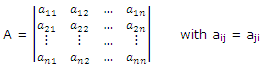# Describe the real quadratic form of a matrix

Publish On: 2019-03-15

Total Post: 752

# Mjay Jollay

Total Post: 0

## ANS: Describe the real quadratic form of a matrix

An expression of the form Σ Σaijxixj, where aij ϵ ℝ and aij = aji, is called a real quadratic form in the variables x1, x2, …., xn. A real quadratic form can be written as XtAX, where X = (x1, x2, …., xn)t and A is a symmetric matrix, (called the matrix of the quadratic form) asFollowing are some facts about a real quadratic form.

A real quadratic form:

(i) is usually denoted by Q(x1, x2, …, xn), where xi are the variables

(ii) has rank r if its matrix has rank r

(iii) has signature m if its matrix has signature m

(iv) is positive definite if Q(x1, x2, …., xn) > 0 for all values of x1, x2, ….., xn

(v) is negative definite if Q(x1, x2, …., xn) < 0 for all values of x1, x2, ….., xn

(vi) is positive semidefinite if Q(x1, x2, …., xn) > 0 for some values of x1, x2, …., xn and zero for other values of x1, x2, …., xn

(vii) is negative semidefinite if Q(x1, x2, …., xn) < 0 for some values of x1, x2, …., xn and zero for other values of x1, x2, …., xn.

(viii) is indefinite if Q(x1, x2, …., xn) ≥ 0 for some values of x1, x2, …., xn and Q(x1, x2, …., xn) < 0 for other values of x1, x2, …., xn.